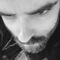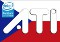# Can I use SQL array as in Dibi?Robin Martinez
Member | 81
+
0
-

Hi,

I love Dibi and use it a lot. I heavily used sql array to feed Dibi such as:

``````\$q = []
array_push('SELECT * FROM %n', \$table);
array_push('WHERE id > %d', \$id);

\$res = dibi::fetchAll(\$q);``````

I guess this is not possible in Nette Database? The only ‘similar’ method I found is:

``````\$q = \$args = [];
\$q[] = "SELECT * FROM ?";
\$args[] = \$table;

\$q[] = "WHERE id > ?";
\$args[] = \$id;

\$res = \$this->db->fetchAll(implode(' ', \$q), ...\$args);``````

Or is there any ‘cleaner’ method? Thanks!CZechBoY
Member | 3608
+
0
-

Why not to use dibi if you know it?Robin Martinez
Member | 81
+
0
-

I just started a new job, where Nette is used. So I'd like to know if I can use old habits :)CZechBoY
Member | 3608
+
0
-

I'm not sure if this work, but try it :D
`->where(\$q, \$args)`